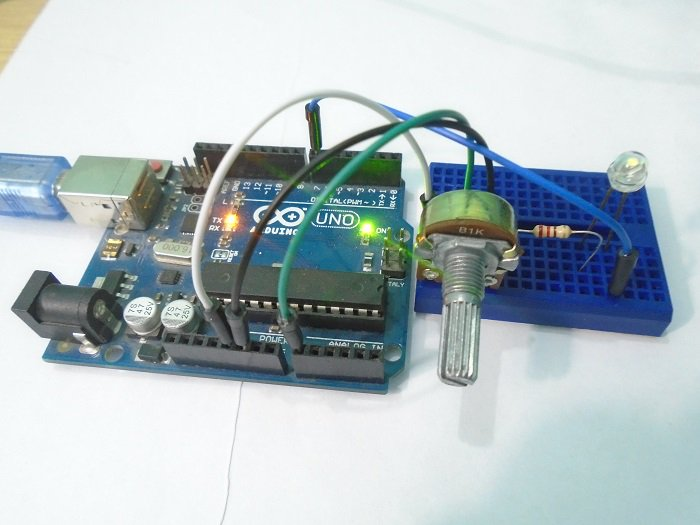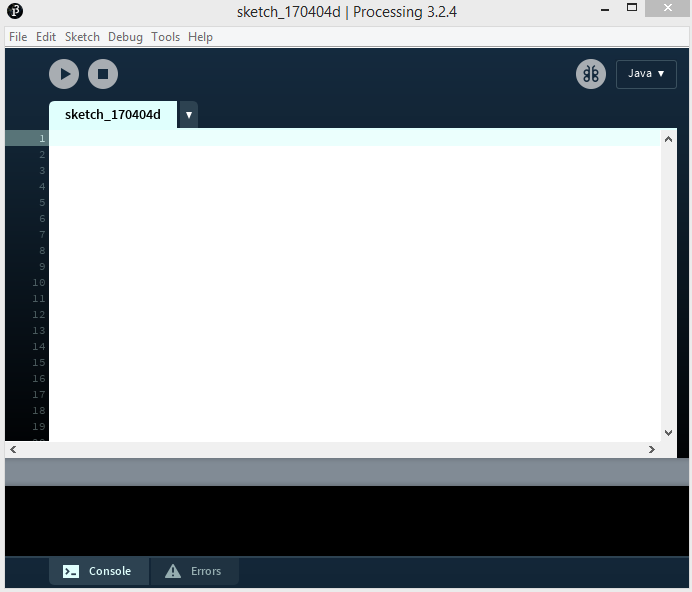Arduino

# How to Make Arduino and Processing IDE CommunicateMarch 21, 2018 by A .
ShareLearn how to connect the Arduino to the Processing IDE so they can communicate with one another.

In this tutorial, we will make the communication between the Arduino and Processing. We will make the communication in both the ways, from the Arduino to Processing and from Processing to the Arduino. We will change the color of the serial window in the Processing IDE through the potentiometer value sent from the Arduino IDE. We will turn the LED ON and OFF depending upon the mouse button pressed in the Processing Serial window.Processing is a great source for creating graphics. The Processing IDE works for a computer like the Arduino IDE works for a micro-controller. The Processing IDE is similar to Arduino in terms of structure. It has setup functions and draw functions like an Arduino has a setup and loop function. The Processing IDE can communicate with the Arduino IDE through serial communication. This way, we can send data from the Arduino to the Processing IDE and also from the Processing IDE to the Arduino.

### Installing Processing### Arduino Circuit Diagram

The connections for this tutorial are very easy. Connect the two end pins of the 1k potentiometer to the 5V and the GND pin of the Arduino. Then connect the middle pin of the potentiometer to the A0 on Arduino. Then connect the positive pin of the LED to pin 7 on the Arduino and the negative pin of the LED to the GND pin through the 220 ohm resistor.### How Does It Work?

The Arduino IDE and the Processing IDE will communicate with each other through serial communication. The Processing IDE has a serial library which makes it easy to communicate with the Arduino.

When we move the potentiometer knob, the Arduino will send a value between 0 and 255 to the Processing IDE. The Processing IDE will then change the color of the serial window according to the movement of the potentiometer knob.

Similarly, when we press the mouse button in the serial window of the Processing IDE, the Processing IDE will send a â€˜1â€™ or â€˜0â€™ depending on the left or right mouse button to the Arduino IDE. The Arduino IDE will then turn the LED ON or OFF according to the button pressed.

### Arduino Code

The Arduino Code is very easy; everything is explained in the code.

``````int led_pin = 7;    // Initializing the LED pin

int pot_pin = A0;   // Initializing the Potentiometer pin

int pot_output;     // Declaring a variable for potentiometer output

void setup ( ) {

pinMode(led_pin, OUTPUT); // Declaring the LED pin as output pin

Serial.begin(9600);       // Starting the serial communication at 9600 baud rate

}

void loop ( ) {

int mapped_output = map (pot_output, 0, 1023, 0, 255); // Mapping the output of potentiometer to 0-255 to be read by the Processing IDE

Serial.println (mapped_output);     // Sending the output to Processing IDE

if (Serial.available ( ) > 0) {   // Checking if the Processing IDE has send a value or not

char state = Serial.read ( );    // Reading the data received and saving in the state variable

if(state == '1')             // If received data is '1', then turn on LED

{

digitalWrite (led_pin, HIGH);

}

if (state == '0') {     // If received data is '0', then turn off led

digitalWrite (led_pin, LOW);

}

}

delay(50);

}``````

### Processing Code

The Processing IDE will accept the data from the Arduino IDE through the Serial communication and will change the color of the serial window according to the data. It will also send â€˜1â€™ or â€˜0â€™ depending on the mouse button pressed. The Processing IDE has a serial library which makes it able to communicate with the Arduino IDE.

``````import processing.serial.*;    // Importing the serial library to communicate with the Arduino

Serial myPort;      // Initializing a vairable named 'myPort' for serial communication

float background_color ;   // Variable for changing the background color

void setup ( ) {

size (500,  500);     // Size of the serial window, you can increase or decrease as you want

myPort  =  new Serial (this, "COM3",  9600); // Set the com port and the baud rate according to the Arduino IDE

myPort.bufferUntil ( '\n' );   // Receiving the data from the Arduino IDE

}

void serialEvent  (Serial myPort) {

background_color  =  float (myPort.readStringUntil ( '\n' ) ) ;  // Changing the background color according to received data

}

void draw ( ) {

background ( 150, 50, background_color );   // Initial background color, when we will open the serial window

if ( mousePressed  &&  ( mouseButton  ==  LEFT ) ) { // if the left mouse button is pressed

myPort.write ( '1' ) ;       // send a '1' to the Arduino IDE

}

if  ( mousePressed  &&  ( mouseButton == RIGHT ) ) {  // if the right mouse button is pressed

myPort.write ( '0' ) ;     // Send a '0' to the Arduino IDE

}

}``````

Now that you've learned some basic Arduino programming, you're ready to tackle some more Arduino projects!

### AuthorA .

For custom projects, hire me at https://www.freelancer.pk/u/Muhammadaqibdutt